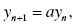# 2.1. MODELING WITH FIRST-ORDER LINEAR HOMOGENOUS DIFFERENCE EQUATIONS WITH CONSTANT COEFFICIENTS

In this section, we investigate situations that are modeled by first-order linear homogenous difference equations with constant coefficients, that is, equations in the form

(2.1)where a is a constant coefficient. We will iterate equation 2.1 with an initial condition to find a numerical solution, and we will also derive an analytical solution of equation 2.1.

## 2.1.1. Model 2.1: Drugs

Assume that the kidneys remove 20% of a drug from the blood every 4 h. Assume that the initial dose of the drug is 200 mg. Let dn denote the amount of drug in the blood after n 4-h periods, and d0 denote the initial amount of drug in the blood.

i. Find a difference equation that represents this situation.
ii. Find the amount of drug after 12 hours.
iii. Iterate the difference equation obtained in part I with the initial condition to find the ordered pairs (n, dn), n = 1, 2, … , 28. Graph it.
iv. Find an analytical solution of the obtained difference equation. Use this solution to find the amount of drug in the blood after 1 day.
v. When will the amount of the drug reach 1 mg?

### Discussion

i. The amount of drug in the blood after (n + 1) 4-h periods, dn+1, equals the amount of drug after n 4-h periods, dn, minus 20% of dn. We obtain
Therefore this situation ...

Get Explorations of Mathematical Models in Biology with MATLAB now with the O’Reilly learning platform.

O’Reilly members experience books, live events, courses curated by job role, and more from O’Reilly and nearly 200 top publishers.